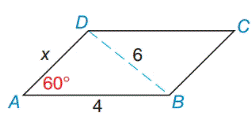Chapter 11.4, Problem 27EElementary Geometry For College St...

7th Edition
Alexander + 2 others
ISBN: 9781337614085

Solutions

Chapter
SectionElementary Geometry For College St...

7th Edition
Alexander + 2 others
ISBN: 9781337614085
Textbook Problem

In Exercises 23 to 28, use a form of the Law of Cosines to find the measure of the indicated side or angle. Angle measures should be found to the nearest degree and lengths of sides to the nearest tenth of a unit.Parallelogram ABCD

To determine

To find:

The value of x the given figure by using the Law of Cosines.

Explanation

Formula:

The Law of Cosines

cosα=b2+c2a22bc

cosβ=a2+c2b22ac

cosγ=a2+b2c22ab

Where α, β, and γ is the acute angle of the triangle, and a, b, and c is the sides of the triangle.

Calculation:

Given,

From the figure,

a=x

b=4

c=6

By the Law of Cosines,

c2=a2+b22abcosγ

(6)2=a2+(4)22(x)(4)cos60°

36=x2+(4)22(x)(4)

Still sussing out bartleby?

Check out a sample textbook solution.

See a sample solution

The Solution to Your Study Problems

Bartleby provides explanations to thousands of textbook problems written by our experts, many with advanced degrees!

Get Started

Find all possible real solutions of each equation in Exercises 3144. x3x23x+3=0

Finite Mathematics and Applied Calculus (MindTap Course List)

Find the domain of the function. a. f(x) = 9x b. f(x) = x+32x2x3

Applied Calculus for the Managerial, Life, and Social Sciences: A Brief Approach

True or False: converges.

Study Guide for Stewart's Single Variable Calculus: Early Transcendentals, 8th